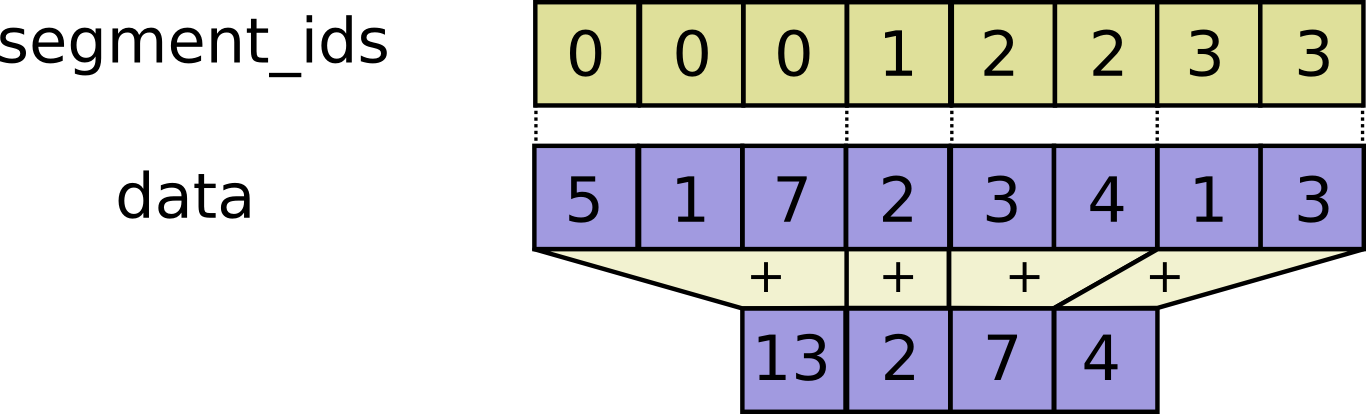# tf.math.segment_sum

Computes the sum along segments of a tensor.

Read the section on segmentation for an explanation of segments.

Computes a tensor such that $$output_i = \sum_j data_j$$ where sum is over j such that segment_ids[j] == i.

If the sum is empty for a given segment ID i, output[i] = 0.#### For example:

c = tf.constant([[1,2,3,4], [4, 3, 2, 1], [5,6,7,8]])
tf.segment_sum(c, tf.constant([0, 0, 1]))
# ==> [[5, 5, 5, 5],
#      [5, 6, 7, 8]]


data A Tensor. Must be one of the following types: float32, float64, int32, uint8, int16, int8, complex64, int64, qint8, quint8, qint32, bfloat16, uint16, complex128, half, uint32, uint64.
segment_ids A Tensor. Must be one of the following types: int32, int64. A 1-D tensor whose size is equal to the size of data's first dimension. Values should be sorted and can be repeated.
name A name for the operation (optional).

A Tensor. Has the same type as data.

[]
[]